## Piecewise Constant Potentials in One Dimension

We now study the physics of several simple potentials in one dimension. First a series of piecewise constant potentials for which the Schrödinger equation is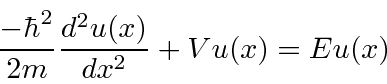orand the general solution, for, can be written as either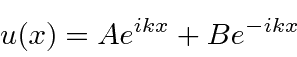or, with. We will also need solutions for the classically forbidden regions where the total energy is less than the potential energy,.with. (Both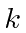andare positive real numbers.) The 1D scattering problems are often analogous to problems where light is reflected or transmitted when it at the surface of glass.

First, we calculate the probability the a particle of energyis reflected by a potential step of height:. We also use this example to understand the probability current.

Second we investigate the square potential well square potential well (forandelsewhere), for the case where the particle is not bound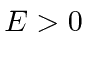. Assuming a beam of particles incident from the left, we need to match solutions in the three regions at the boundaries at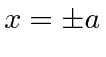. After some difficult arithmetic, the probabilities to be transmitted or reflected are computed. It is found that the probability to be transmitted goes to 1 for some particular energies.This type of behavior is exhibited by electrons scattering from atoms. At some energies the scattering probability goes to zero.

Third we study the square potential barrier (forandelsewhere), for the case in which. Classically the probability to be transmitted would be zero since the particle is energetically excluded from being inside the barrier. The Quantum calculation gives the probability to be transmitted through the barrier to be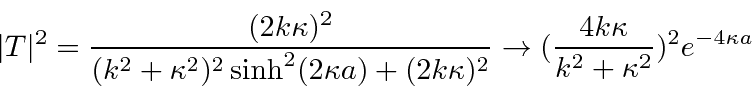whereand. Study of this expression shows that the probability to be transmitted decreases as the barrier get higher or wider. Nevertheless, barrier penetration is an important quantum phenomenon.

We also study the square well for the bound state case in which. Here we need to solve a transcendental equation to determine the bound state energies. The number of bound states increases with the depth and the width of the well but there is always at least one bound state.

Jim Branson 2013-04-22